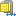### Formulas

#### Write formula

No problem to create Excel formulas with Pandas, but use these tips below.

• As you will use simple and double quote in your formulas, frame your string with triple quotes.
• Translate in english all Excel keywords (IF, CHAR, SUBSTITUTE...).
• Use a simple comma to separate your parameters (english...).
```df.sort_values(by=['Your Id'], ascending=True, inplace=True)
df = df.reset_index(drop=True)```
• Start the index with a special value (to start with 2, as usual in Excel):
`df.index = df.index + 2`
• Then use the index as a string in your formula:
`'''...''' + df.index.astype(str) + '''...'''`
• Do not add other sorts after the formulas creation, it causes index issues and impacts your formulas.

Sometime you want read formula as string. If the Excel file was created by Excel himself, no problem, just use `data_only=True`:

```wb = openpyxl.load_workbook('My file.xlsx', data_only = True)
ws = wb['My tab']
df = pd.DataFrame(ws.values)
But if the file, and the formulas, was created with Python, so the Excel cache do not know your formulas and... oups!
So just open and close your file before to use `data_only=True`:
```import xlwings
...
excel_app = xlwings.App(visible=False)
excel_book = excel_app.books.open('My file.xlsx')
excel_book.save()
excel_book.close()
excel_app.quit()```

### Conditional formatting

#### If a number is equal to, in just one cell

Here we add in one cell (B3) a rule to fill it in blue if it equals to 2:

```import openpyxl
from openpyxl import styles, formatting

myFile = 'C:/Path/test.xlsx'

myDF.to_excel(myFile, index=False, sheet_name='Tab 1')

worksheet = workbook['Sheet1']

myBlue = styles.PatternFill(start_color='0088cc', end_color='0088cc', fill_type='solid')

workbook.save(myFile)```

#### If a number is greater than, in whole column

Same as above, we just change the operator with `greaterThan` and we target the whole column B as Excel did, with  `\$1:\$1048576`:

```import openpyxl
from openpyxl import styles, formatting

myFile = 'C:/Path/test.xlsx'

myDF.to_excel(myFile, index=False, sheet_name='Tab 1')

worksheet = workbook['Sheet1']

myBlue = styles.PatternFill(start_color='0088cc', end_color='0088cc', fill_type='solid')

workbook.save(myFile)```

#### Operators

The above method supports several operators:

`{'beginsWith', 'endsWith', 'notBetween', 'notContains', 'lessThan', 'greaterThanOrEqual', 'notEqual', 'between', 'lessThanOrEqual', 'containsText', 'greaterThan', 'equal'}`

#### If contains a string

For match with strings it is a little specific, with redundant code and building the `column_slot` ourself, using the size of our dataframe `+1` :

Here for example we fill in red the cells from A column when contain the string CSS.

```import pandas as pd
import openpyxl
from openpyxl import styles, formatting
from openpyxl.styles.differential import DifferentialStyle
from openpyxl.formatting.rule import Rule

myFile = 'C:/Path/test.xlsx'

myDF.to_excel(myFile, index=False, sheet_name='Tab 1')

worksheet = workbook['Sheet1']

myRed = styles.PatternFill(start_color='ffc7ce', end_color='ffc7ce', fill_type='solid')

column_slot = 'A2:A'+str(myDF.shape+1)

styleRed = DifferentialStyle(fill=myRed)
rule = Rule(type='containsText', operator='containsText', text='css', dxf=styleRed)
rule.formula = ['SEARCH("css",' + column_slot + ")"]

workbook.save(myFile)```

### Fix Excel percentage

When your data comes from an Excel file and contains percentages, something like `dtype` (search it in Miscellaneous chapter) will be non-efficient, because Excel stores percentages as numbers (indices between 0 and 1). The displaying with % is just a view. Actually 35% will be stored as 0.3 for example.

In addition, it is possible that these percentages are melted with true strings or numbers in the same columns, and sometimes with or without decimal ... Argghhh 🥵

But just relax:

```import re
...
df[MyField] = df[MyField].apply(lambda x: str(round(float(x) * 100, 2)).rstrip('0').rstrip('.') + ' %' if re.search(r'^0\.\d+\$|^0\$|^1\$',x) else x)```
Attention
If the Excel file is wrong builded, melting true numbers between 0 and 1 with percentages, they will be converted. So maybe add red alerts to manage these special cases.France-Departements-Deformation.zip [France-Departements-Deformation] 335 Kosimple_countries.zip [simple_countries] 1880 Ko